### Aluminium Guide

UNDERSTANDING OF ALUMINUM# The mechanical properties of aluminum

## What are mechanical properties?

mechanical aluminum properties, as well as other materials - are properties, are associated with the elastic and inelastic material reacting on the applied load, including, the relationship between stress and strain. Examples of mechanical properties are:

• modulus of elasticity (in tension, compressive, at shift)
• tensile strength (tensile, compressive, at shift)
• yield stress
• endurance strength
• elongation (relative) at break
• hardness.

Mechanical properties are often mistakenly attributed to physical properties.

Mechanical properties of materials, including, aluminum and its alloys, which are obtained by testing tensile material, for example, tensile modulus, Tensile strength, tensile yield strength and elongation are called tensile properties.

## Elastic modulus

Elastic modulus, which is often called Young's Modulus - is the ratio of the voltage, is applied to the material, to the corresponding strain in the range, when they are directly proportional to each other.

There are three types of stress, and accordingly three types of the elastic moduli of the material for any, including for aluminum:

• tensile modulus
• modulus of compression
• shear modulus (shear modulus).

Table – Tensile moduli of aluminum and other metals 

##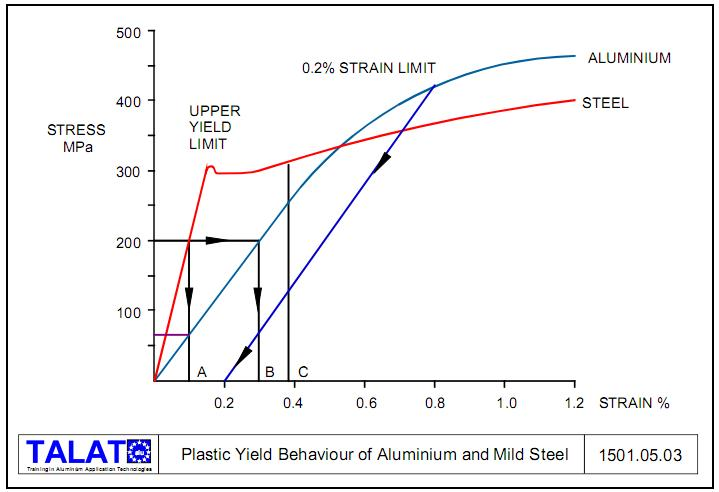Figure 1 – Tensile curves of aluminum and mild steel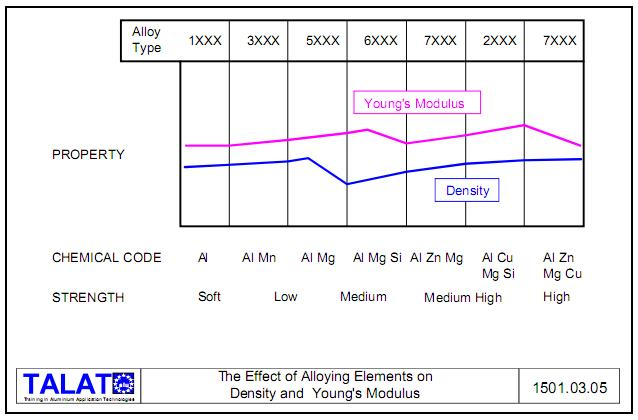Figure 2 – The influence of alloying elements in aluminum alloys on their density t modulus of elasticity 

## Tensile strength

The ratio of the maximum load before failure of the sample when tested in tension at the initial sample cross-sectional area. The terms "tensile strength" as used and "Tensile".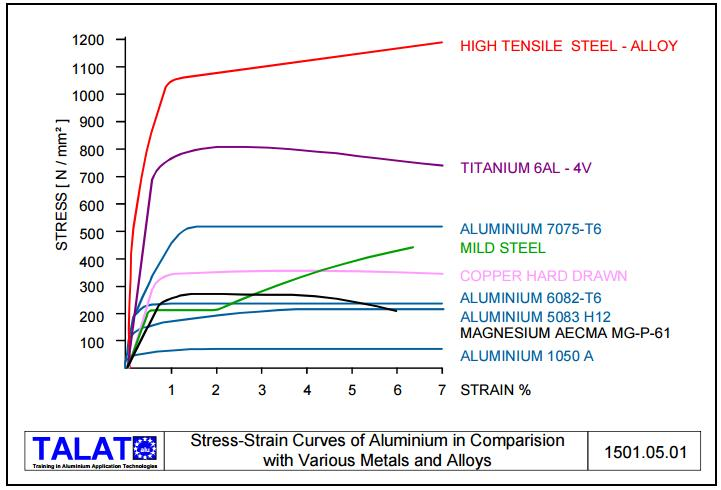Figure 3 – Tensile curves of aluminum in comparison with various metals and alloys 

## yield strength

Voltage, which is necessary to achieve a given small plastic deformation in the aluminum or other material with a uniaxial tensile or compressive load.

If the plastic deformation under tensile load is defined as 0,2 %, then it uses the term "yield stress 0,2 %» (Rp0,2).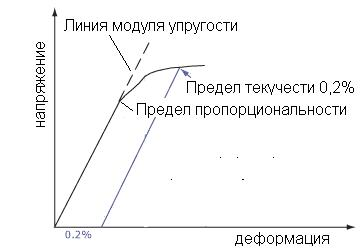Figure 4 – A typical stress-strain diagram
for aluminum alloys

## Elongation (at break)

Often referred to as "elongation". Increasing the distance between the two marks on the test specimen, which occurs as a result of deformation of the sample in tension to the gap between these tags.

The magnitude of the elongation depends on the cross-sectional dimensions of the sample. for instance, elongation value, which is obtained during the test aluminum sheet sample to be lower for the thin sheet, than for the plate. The same applies to the aluminum extrusions.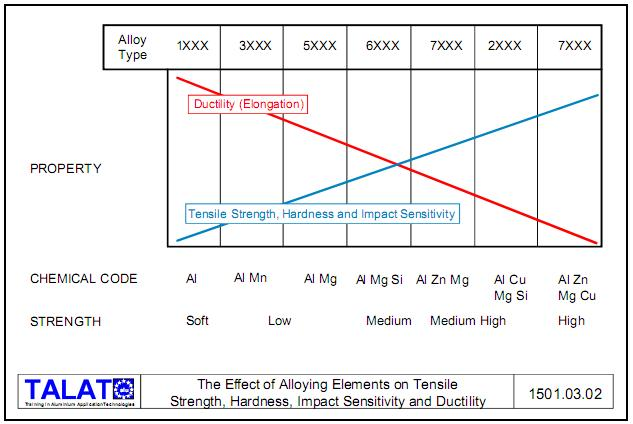Figure 5 – Effect of alloying elements on strength properties and elongation 

### elongation A

Elongation percentage after sample rupture in the initial distance between marks 5,65 · √ S0, where S0 - initial cross-sectional area of ​​the test sample. Dated this designation value A5 It is currently used. A similar magnitude in the Russian-language documents is designated δ5.

It is easy to check, that for round samples this distance between the original marks is calculated as 5 d.

### elongation A50mm

Elongation percentage after rupture of the sample relative to the original length between the marks 50 mm and constant original width of the test piece (usually 12,5 mm). In the US, the separation distance between the marks in 2 inch, i.e 50,8 mm.

## shear strength

Maximum specific voltage, that is, the maximum load, divided by the initial cross-sectional area, material that withstands at a shear test. Shear strength is usually about 60 % of tensile strength.

Shear strength is an important characteristic quality rivets, including, aluminum.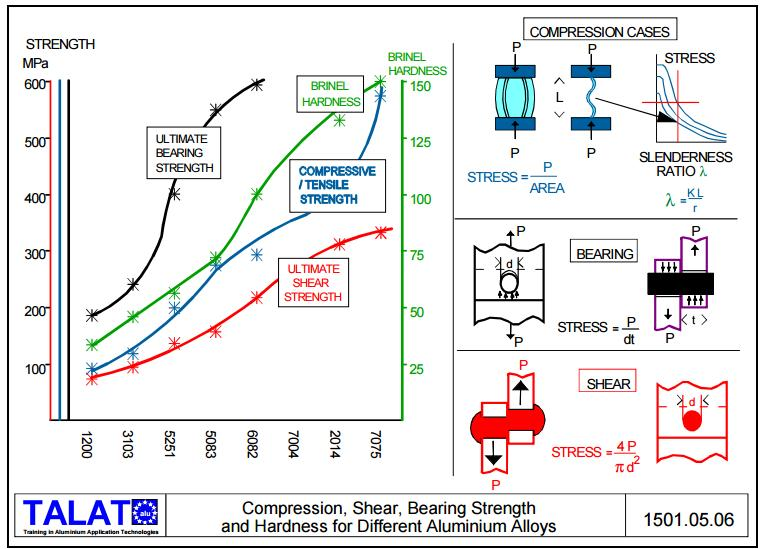Figure 6 – Compressive strength, Shear strength, bearing strength and
hardness of various aluminum alloys 

## Poisson's ratio

The ratio between the longitudinal elongation and cross-sectional reduction in uniaxial testing. For aluminum and aluminum alloys, all in all states of the Poisson's ratio is usually 0,33 .

## Hardness

Resistance to plastic deformation of the metal, usually measured by indentation.

### Brinell hardness (HB)

Resistance to penetration of a spherical indentor under standardized conditions.

For aluminum and aluminum alloys, the HB hardness is approximately 0.3 Rm, where Rm - ultimate tensile strength, expressed in MPa .

If applied tungsten carbide indenter, the designation applies HBW.

### Vickers hardness (HV)

Penetration resistance diamond indenter in the form of a square pyramid under standardized conditions. The HV hardness is approximately equal to 1.10 · HB .

## Fatigue

The tendency of the metal to break down during prolonged cyclical voltage, which is much lower tensile strength.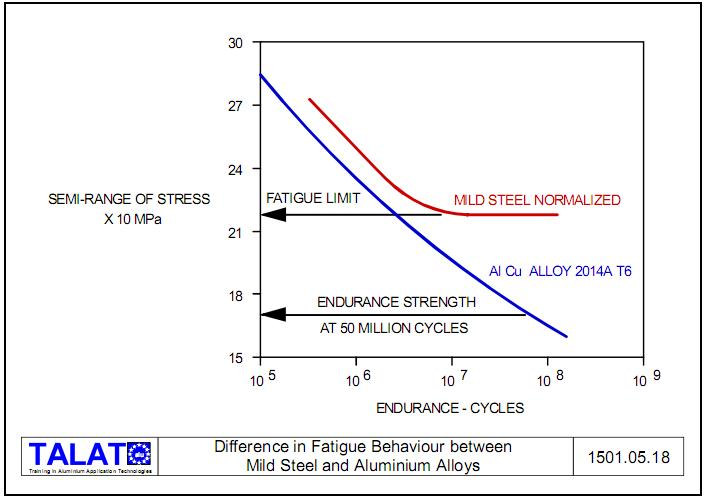Figure 7 – Difference in fatigue behavior of low carbon steel and aluminum alloys 

### fatigue strength

The maximum amplitude of the voltage, which can withstand the product for a given number of cycles. Usually expressed as a voltage amplitude, which gives a 50% probability of failure after a given number of loading cycles .

### fatigue strength

limiting stress, below which the material will withstand a given number of stress cycles .

## The mechanical properties of aluminum and aluminum alloy

The tables below  show typical mechanical properties of aluminum and aluminum alloys:

• tensile strength
• yield strength in tension
• Tensile elongation
• fatigue strength
• hardness
• elastic modulus

Mechanical properties are presented separately:These mechanical properties – typical. It means, that they are suitable only for comparative purposes, but not for engineering calculations. In most cases, they are average values ​​for the different sizes of products, their forms and methods of manufacture.

Source:

1. Materials Aluminum Association Germany
2. Global Advisory Group GAG – Guidance “Terms and Definitions” – 2011-01
3. Aluminium and Aluminium Alloys. – ASM International, 1993.
4. TALAT 1501